Next: Fixed Point Free Automorphisms Up: Complexity Previous: Basic Issues

## Endomorphism Graphs

Recall that a graph   is a pair G=(V,E) of a set V of vertices   and a set E of doubleton subsets of V, called edges.     The induced subgraph of a graph G=(V,E) with vertex set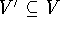is the graph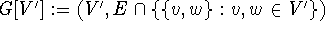. Unless otherwise stated all subgraphs will be induced subgraphs.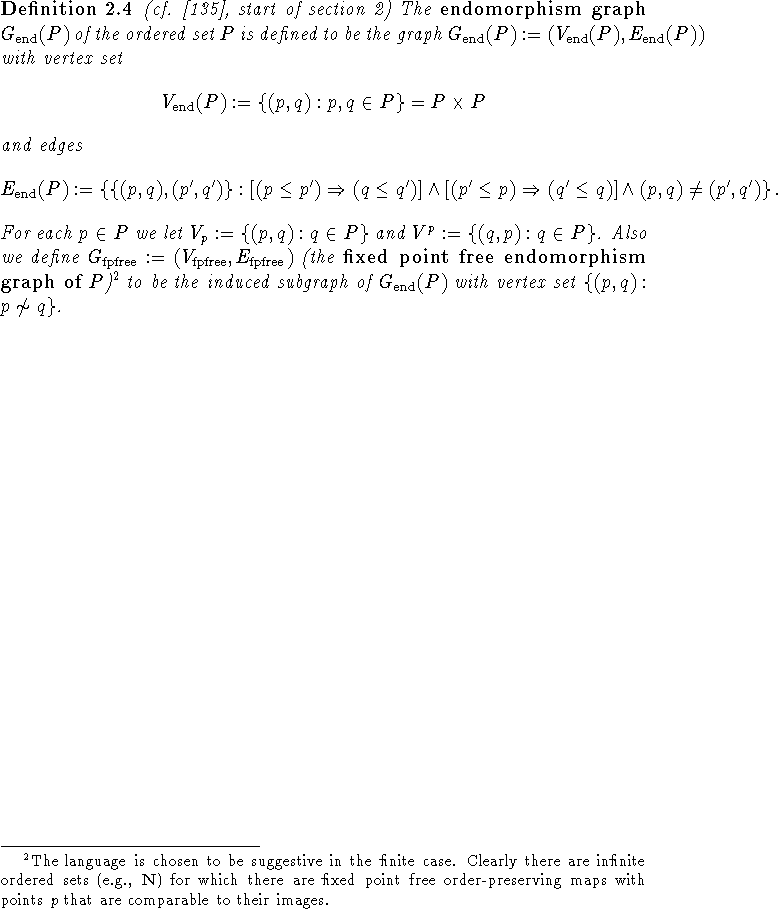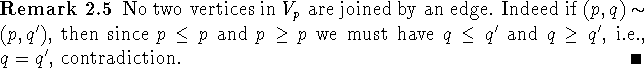The choice of language can be justified by the following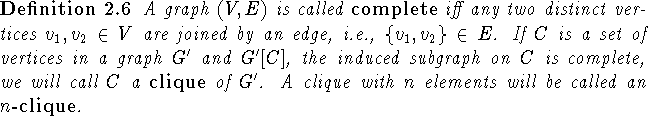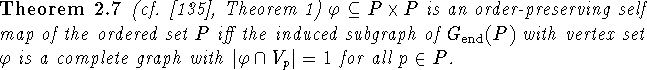Proof: ``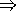": If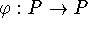is an order-preserving self map then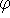is in particular well-defined and thus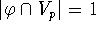for all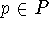. Now let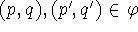. Then sinceis order-preserving the logical statement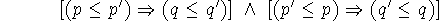is true and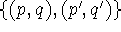is an edge in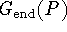.
``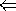": Let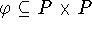be such that the induced subgraph ofwith vertex setis a complete graph withfor all. The latter immediately shows thatis well-defined, i.e., a function. Now let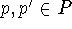with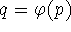,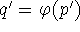. If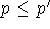, then, sinceis a true logical statement, we have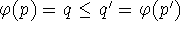. Thusis order-preserving. \

Based on criterion 2.7 various kinds of order-preserving self maps can be identified in the endomorphism graph.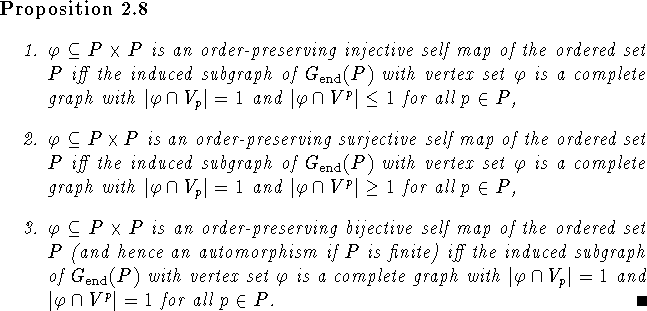Theorem 2.7 is applicable to infinite ordered sets. For the computationally more interesting finite case the conditionfor all p can be replaced with the demand thathas |P| vertices. With regards to fixed point free order-preserving maps on finite ordered sets we obtain: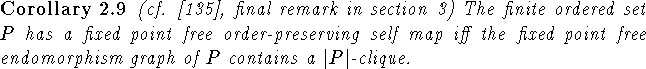Proof: This follows directly from the analogue of Theorem 2.7 for fixed point free order-preserving self maps. Just note that by Remark 2.5 any complete subgraph of the endomorphism graph has at most one vertex in each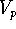. \

In order to decide if a finite ordered set P has a fixed point free order-preserving self map one would thus need to decide if the fixed point free endomorphism graph has a |P|-clique. As mentioned in  there currently are algorithms that determine the maximal size of a clique in a graph in acceptable time for graphs with up to 400 to 1000 vertices. As the fixed point free endomorphism graph of P has at most |P|(|P|-1) vertices and normally actually fewer than that, this means the fixed point property can be determined through the fixed point free endomorphism graph for ordered sets with up to 20 (guaranteed) to (possibly) 50 or more points.
To decide if a finite ordered set P has a fixed point free automorphism one would need to decide if the fixed point free endomorphism graph has a complete induced subgraph as described in part 3 of Proposition 2.8 and Corollary 2.9. To get a completely analogous formulation consider: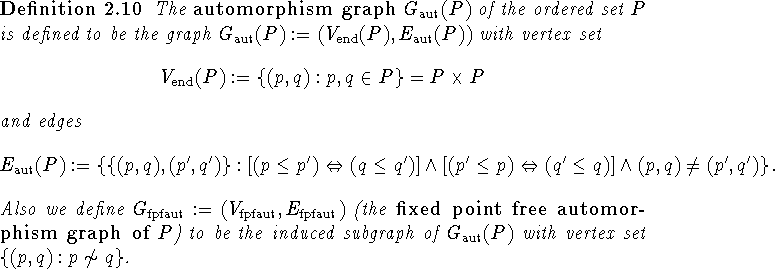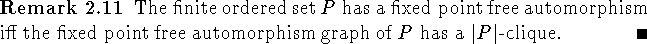Next: Fixed Point Free Automorphisms Up: Complexity Previous: Basic Issues

Bernd.S.W.Schroder